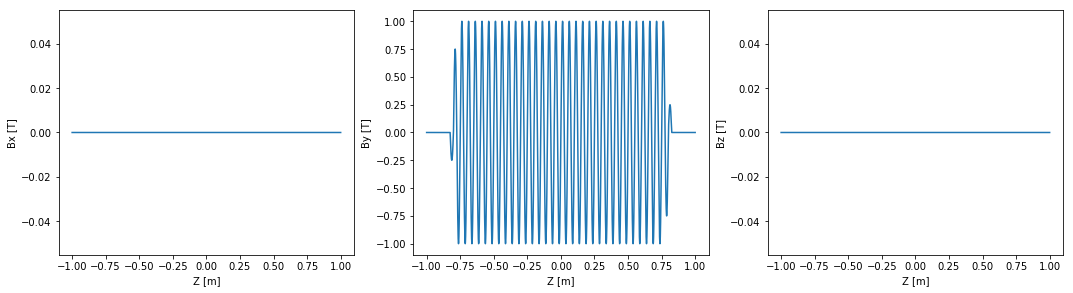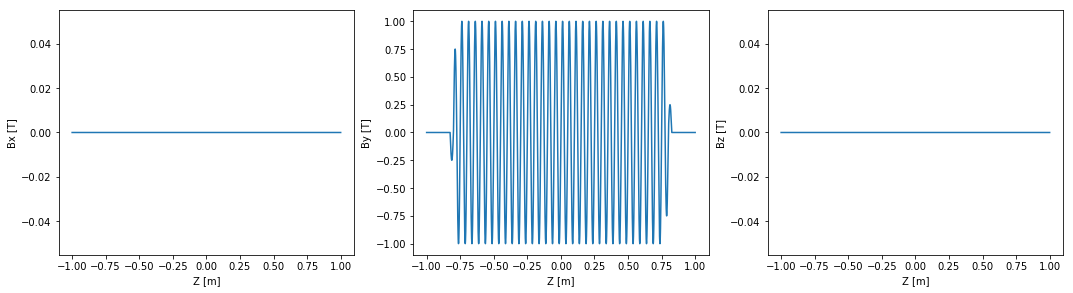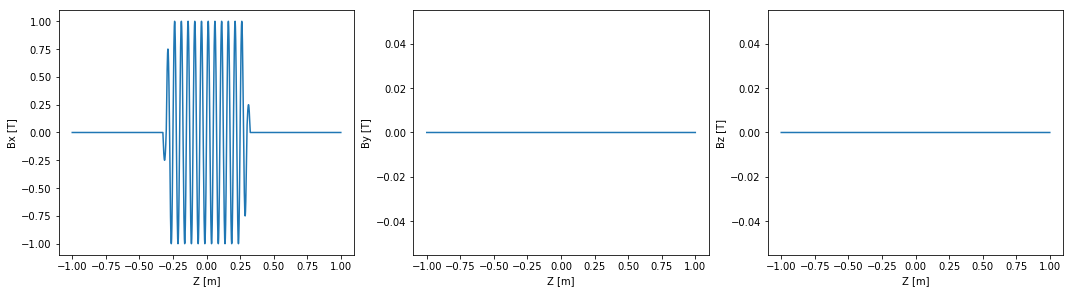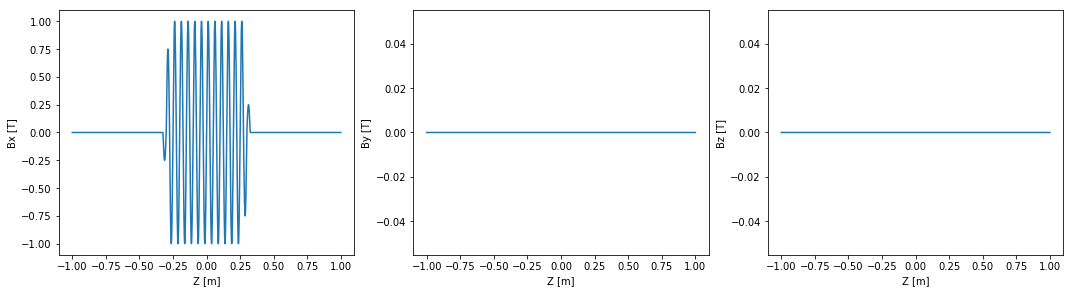# Importing Field Data¶

OSCARS supports several basic data formats for importing. We are interested in supporting more.

One basic format ('OSCARS1D') is where you have the magnetic field (1, 2, or 3D) given as a function of position and your file format lists rows of (for instance) 'Z Bx By Bz'. In this case, specify X, Y, or Z, and whichever of the B's you have in the format field: iformat='Z Bx By Bz'.

OSCARS format: Has 10 lines of header information, followed by lines consisting of 'Bx By Bz'. You must specify iformat='OSCARS'. The header is as follows:

• Comment line
• Initial X position
• Step size in X
• Number of points in X
• Initial Y position
• Step size in Y
• Number of points in Y
• Initial Z position
• Step size in Z
• Number of points in Z

OSCARS also supports iformat='SPECTRA' and iformat='SRW'. See the All About Magnetic Fields tutorial for mroe information.

In :
# matplotlib plots inline
%matplotlib inline

# Import the OSCARS SR module
import oscars.sr

# Import OSCARS plots (matplotlib)
from oscars.plots_mpl import *

OSCARS v2.1.8 - Open Source Code for Advanced Radiation Simulation
Brookhaven National Laboratory, Upton NY, USA
http://oscars.bnl.gov
oscars@bnl.gov

In :
# Create a new OSCARS object.  Default to 8 threads and always use the GPU if available


## OSCARS1D Format¶

In :
# To illustrate the basic 1D format let's create a data file, then import it.
# It will be plotted before and after the import

# Create an undulator field
osr.clear_bfields()
osr.add_bfield_undulator(bfield=[0, 1, 0], period=[0, 0, 0.05], nperiods=31)
plot_bfield(osr)

# Now write the field to a file
osr.write_bfield(
ofile='GettingStarted_OSCARS1D.dat',
oformat='OSCARS1D Z By Bx Bz',
zlim=[-1, 1],
nz=5000
)

# Clear fields and import the field from the file created above
osr.clear_bfields()
osr.add_bfield_file(ifile='GettingStarted_OSCARS1D.dat', iformat='OSCARS1D Z By Bx Bz')
plot_bfield(osr)## OSCARS Format¶

In :
# To illustrate the basic 3D format let's create a data file, then import it.
# It will be plotted before and after the import

# Create an undulator field
osr.clear_bfields()
osr.add_bfield_undulator(bfield=[1, 0, 0], period=[0, 0, 0.050], nperiods=11)
plot_bfield(osr)

# Now write the field to a file
osr.write_bfield(
ofile='GettingStarted_OSCARS.dat',
oformat='OSCARS',
xlim=[-1, 1],
nx=2,
ylim=[-1, 1],
ny=2,
zlim=[-1, 1],
nz=5000
)

# Clear fields and import the field from the file created above
# You can also scale position, in case your input is not in [m]
osr.clear_bfields()